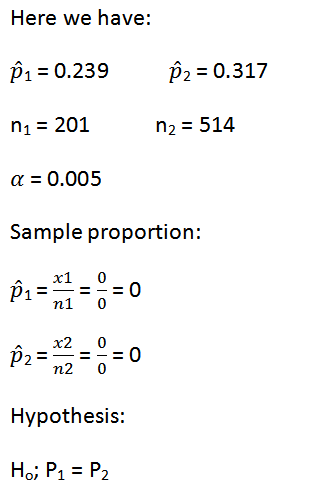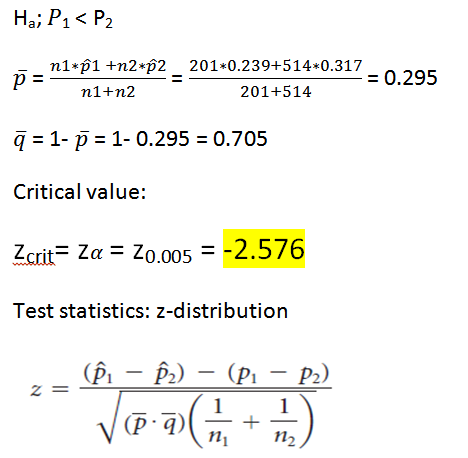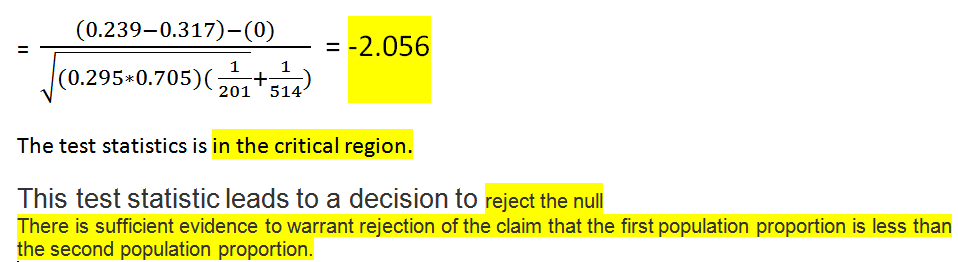Question

# You wish to test the following claim (Ha) at a significance level of α=0.005.       Ho:p1=p2...

You wish to test the following claim (Ha) at a significance level of α=0.005.

Ho:p1=p2
Ha:p1<p2

You obtain 23.9% successes in a sample of size n1=201 from the first population. You obtain 31.7% successes in a sample of size n2=514 from the second population. For this test, you should NOT use the continuity correction, and you should use the normal distribution as an approximation for the binomial distribution.

What is the critical value for this test? (Report answer accurate to three decimal places.)
critical value =

What is the test statistic for this sample? (Report answer accurate to three decimal places.)
test statistic =

The test statistic is...

• in the critical region
• not in the critical region

This test statistic leads to a decision to...

• reject the null
• accept the null
• fail to reject the null

As such, the final conclusion is that...

• There is sufficient evidence to warrant rejection of the claim that the first population proportion is less than the second population proportion.
• There is not sufficient evidence to warrant rejection of the claim that the first population proportion is less than the second population proportion.
• The sample data support the claim that the first population proportion is less than the second population proportion.
• There is not sufficient sample evidence to support the claim that the first population proportion is less than the second population proportion.#### Earn Coins

Coins can be redeemed for fabulous gifts.

Similar Homework Help Questions
• ### You wish to test the following claim (H1) at a significance level of α=0.10.       Ho:p1=p2       H1:p1<p2...

You wish to test the following claim (H1) at a significance level of α=0.10.       Ho:p1=p2       H1:p1<p2 You obtain a sample from the first population with 423 successes and 41 failures. You obtain a sample from the second population with 294 successes and 25 failures. What is the critical value for this test? (Report answer accurate to three decimal places.) critical value = What is the test statistic for this sample? (Report answer accurate to three decimal places.) test statistic =...

• ### You wish to test the following claim (Ha) at a significance level of α=0.10.       Ho:p1=p2 &n...

You wish to test the following claim (Ha) at a significance level of α=0.10.       Ho:p1=p2       Ha:p1<p2 You obtain 13.3% successes in a sample of size n1=226 from the first population. You obtain 21.4% successes in a sample of size n2=541 from the second population. For this test, you should NOT use the continuity correction, and you should use the normal distribution as an approximation for the binomial distribution. What is the test statistic for this sample? (Report answer...

• ### You wish to test the following claim (HaHa) at a significance level of α=0.005.       Ho:p1=p2...

You wish to test the following claim (HaHa) at a significance level of α=0.005.       Ho:p1=p2       Ha:p1>p2 You obtain 106 successes in a sample of size n1=522 from the first population. You obtain 33 successes in a sample of size n2=270 from the second population. For this test, you should NOT use the continuity correction, and you should use the normal distribution as an approximation for the binomial distribution. What is the test statistic for this sample? (Report answer...

• ### You wish to test the following claim (HaHa) at a significance level of α=0.10       Ho:p1=p2       Ha:p1>p2...

You wish to test the following claim (HaHa) at a significance level of α=0.10       Ho:p1=p2       Ha:p1>p2 You obtain 37 successes in a sample of size 616 from the first population. You obtain 19 successes in a sample of size 603 from the second population. What is the test statistic for this sample? (Report answer accurate to three decimal places.) Test statistic = What is the p-value for this sample? (Report answer accurate to four decimal places.) p-value = The p-value...

• ### You wish to test the following claim (HaHa) at a significance level of α=0.01α=0.01.       Ho:p1=p2Ho:p1=p2       Ha:p1>p2Ha:p1>p2...

You wish to test the following claim (HaHa) at a significance level of α=0.01α=0.01.       Ho:p1=p2Ho:p1=p2       Ha:p1>p2Ha:p1>p2 You obtain 212 successes in a sample of size 450 from the first population. You obtain 74 successes in a sample of size 226 from the second population. What is the test statistic for this sample? (Report answer accurate to three decimal places.) Test statistic = What is the p-value for this sample? (Report answer accurate to four decimal places.) p-value = The p-value...

• ### You wish to test the following claim (HaHa) at a significance level of α=0.002α=0.002.       Ho:p1=p2Ho:p1=p2       Ha:p1<p2Ha:p1<p2...

You wish to test the following claim (HaHa) at a significance level of α=0.002α=0.002.       Ho:p1=p2Ho:p1=p2       Ha:p1<p2Ha:p1<p2 You obtain 19.6% successes in a sample of size n1=588n1=588 from the first population. You obtain 26.4% successes in a sample of size n2=277n2=277from the second population. For this test, you should NOT use the continuity correction, and you should use the normal distribution as an approximation for the binomial distribution. What is the test statistic for this sample? (Report answer accurate to three...

• ### You wish to test the following claim (Ha Ha ) at a significance level of α=0.05...

You wish to test the following claim (Ha Ha ) at a significance level of α=0.05 α=0.05 . Ho:p1=p2 Ho:p1=p2 Ha:p1>p2 Ha:p1>p2 You obtain 82.6% successes in a sample of size n1=447 n1=447 from the first population. You obtain 75.2% successes in a sample of size n2=472 n2=472 from the second population. For this test, you should NOT use the continuity correction, and you should use the normal distribution as an approximation for the binomial distribution. The test statistic's value...

• ### You wish to test the following claim (Ha Ha ) at a significance level of α=0.05...

You wish to test the following claim (Ha Ha ) at a significance level of α=0.05 α=0.05 . Ho:p1=p2 Ho:p1=p2 Ha:p1>p2 Ha:p1>p2 You obtain 82.6% successes in a sample of size n1=447 n1=447 from the first population. You obtain 75.2% successes in a sample of size n2=472 n2=472 from the second population. For this test, you should NOT use the continuity correction, and you should use the normal distribution as an approximation for the binomial distribution. The test statistic's value...

• ### You wish to test the following claim (H1H1) at a significance level of α=0.02α=0.02.       Ho:p1=p2Ho:p1=p2       H1:p1<p2H1:p1<p2...

You wish to test the following claim (H1H1) at a significance level of α=0.02α=0.02.       Ho:p1=p2Ho:p1=p2       H1:p1<p2H1:p1<p2 You obtain 33.7% successes in a sample of size n1=718n1=718 from the first population. You obtain 42.5% successes in a sample of size n2=414n2=414 from the second population. What is the test statistic for this sample? (Report answer accurate to three decimal places.) test statistic = What is the p-value for this sample? (Report answer accurate to four decimal places.) p-value = The p-value...

• ### You wish to test the following claim (H a) at a significance level of α = 0.02.

You wish to test the following claim (HaHa) at a significance level of α=0.02α=0.02.      Ho:p1=p2Ho:p1=p2      Ha:p1<p2Ha:p1<p2You obtain 380 successes in a sample of size n1=641n1=641 from the first population. You obtain 179 successes in a sample of size n2=259n2=259 from the second population. For this test, you should NOT use the continuity correction, and you should use the normal distribution as an approximation for the binomial distribution.What is the test statistic for this sample? (Report answer accurate to three decimal places.)test statistic = What is the p-value for...